# Bihar Board 12th Maths Objective Answers Chapter 3 Matrices

Bihar Board 12th Maths Objective Questions and Answers

## Bihar Board 12th Maths Objective Answers Chapter 3 Matrices

Question 1.(b) $$\left[\begin{array}{ccc} 0 & -1 / 3 & -1 / 2 \\ 1 / 3 & 0 & -1 / 5 \\ 1 / 2 & 1 / 5 & 0 \\ 3 / 5 & 1 / 3 & 1 / 7 \end{array}\right]$$

Question 2.
The matrix A = $$\left[\begin{array}{ll} 0 & 1 \\ 1 & 0 \end{array}\right]$$ is a
(a) unit matrix
(c) symmetric matrix
(b) diagonal matrix
(d) skew-symmetric matrix
(d) skew-symmetric matrixQuestion 3.
If $$\left[\begin{array}{cc} x+y & 2 x+z \\ x-y & 2 z+w \end{array}\right]=\left[\begin{array}{cc} 4 & 7 \\ 0 & 10 \end{array}\right]$$, then the values of x, y, z and w respectively are
(a) 2, 2, 3, 4
(b) 2, 3, 1, 2
(c) 3, 3, 0, 1
(d) None of these
(a) 2, 2, 3, 4

Question 4.then find the values of a, b, c, x, y and z respectively
(a) -2, -7, -1, -3, -5, 2
(b) 2, 7, 1, 3, 5, -2
(c) 1, 3, 4, 2, 8, 9
(d) -1, 3, -2, -7, 4, 5
(a) -2, -7, -1, -3, -5, 2

Question 5.
The order of the single matrix obtained from(a) 2 × 3
(b) 2 × 2
(c) 3 × 2
(d) 3 × 3
(d) 3 × 3

Question 6.
$$A=\left[\begin{array}{ll} 1 & -1 \\ 2 & -1 \end{array}\right], B=\left[\begin{array}{ll} x & 1 \\ y & -1 \end{array}\right]$$ and (A + B)2 = A2 + B2, then x + y =
(a) 2
(b) 3
(c) 4
(d) 5
(d) 5Question 7.
If A = $$\left[\begin{array}{lll} 2 & 2 & 1 \\ 1 & 3 & 1 \\ 1 & 2 & 2 \end{array}\right]$$, then A4 – 24 (A – I) =
(a) 5I + A
(b) 5I – A
(c) 5I
(d) 6I
(b) 5I – A

Question 8.
If A is an m × n matrix such that AB and BA are both defined, then B is a
(a) m × n matrix
(b) n × m matrix
(c) n × n matrix
(d) m × n matrix
(b) n × m matrix

Question 9.
If $$\left[\begin{array}{ll} 1 & 2 \\ 3 & 4 \end{array}\right]$$, then A2 – 5A is equal to
(a) 2I
(b) 3I
(c) -2I
(d) null matrix
(a) 2I

Question 10.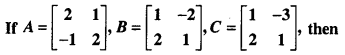(a) A + B = B + A and A + (B + C) = (A + B) + C
(b) A + B = B + A and AC = BC
(c) A + B = B + A and AB = BC
(d) AC = BC and A = BC
(a) A + B = B + A and A + (B + C) = (A + B) + C

Question 11.(a) α = a2 + b2, β = ab
(b) α = a2 + b2, β = 2ab
(c) α = a2 + b2, β = a2 – b2
(d) α = 2ab, β = a2 + b2
(b) α = a2 + b2, β = 2ab

Question 12.
If A = $$\left[\begin{array}{lll} 1 & 2 & x \\ 0 & 1 & 0 \\ 0 & 0 & 1 \end{array}\right]$$ and B = $$\left[\begin{array}{ccc} 1 & -2 & y \\ 0 & 1 & 0 \\ 0 & 0 & 1 \end{array}\right]$$ and AB = I3, then x + y equals
(a) 0
(b) -1
(c) 2
(d) None of these
(a) 0Question 13.
If A = $$\left[\begin{array}{ll} 1 & 2 \\ 2 & 1 \end{array}\right]$$ and f(x) = (1 + x) (1 – x), then f(a) is(a) $$-4\left[\begin{array}{ll} 1 & 1 \\ 1 & 1 \end{array}\right]$$

Question 14.
If A = $$\left[\begin{array}{ll} 1 & 3 \\ 3 & 4 \end{array}\right]$$ and A2 – KA – 5I = 0, then K =
(a) 5
(b) 3
(c) 7
(d) None of these
(a) 5

Question 15.(b) $$\left[\begin{array}{cc} -3 & 10 \\ -2 & 7 \end{array}\right]$$

Question 16.
If matrix A = $$\left[\begin{array}{lll} a & b & c \\ b & c & a \\ c & a & b \end{array}\right]$$ where a, b, c are real positivenumbers, abc = 1 and ATA = I, then the value of a3 + b3 + c3 is
(a) 1
(b) 2
(c) 3
(d) 4
(d) 4Question 17.
Find the values of x, y, z respectively if the matrix $$A=\left[\begin{array}{ccc} 0 & 2 y & z \\ x & y & -z \\ x & -y & z \end{array}\right]$$ satisfy the equation ATA = I3.
(a) $$\frac{1}{\sqrt{2}} \cdot \frac{1}{\sqrt{6}}, \frac{1}{\sqrt{3}}$$
(b) $$\frac{-1}{\sqrt{2}}, \frac{-1}{\sqrt{6}}, \frac{-1}{\sqrt{3}}$$
(c) Both (a) and (b)
(d) None of these
(c) Both (a) and (b)

Question 18.
If $$A=\left[\begin{array}{cc} \cos x & -\sin x \\ \sin x & \cos x \end{array}\right]$$, find AAT.
(a) Zero Matrix
(b) I2
(c) $$\left[\begin{array}{ll} 1 & 1 \\ 1 & 1 \end{array}\right]$$
(d) None of these
(b) I2

Question 19.
If $$A=\left[\begin{array}{ccc} 0 & -1 & 2 \\ 1 & 0 & 3 \\ -2 & -3 & 0 \end{array}\right]$$, then A + 2AT equals
(a) A
(b) -AT
(c) AT
(d) 2A2
(c) AT

Question 20.
For any square matrix A, AAT is a
(a) unit matrix
(b) symmetric matrix
(c) skew-symmetric matrix
(d) diagonal matrix
(b) symmetric matrixQuestion 21.
If A and B are symmetric matrices of the same order, then
(a) AB is a symmetric matrix
(b) A – Bis askew-symmetric matrix
(c) AB + BA is a symmetric matrix
(d) AB – BA is a symmetric matrix
(c) AB + BA is a symmetric matrix

Question 22.
If $$A=\left[\begin{array}{cc} 3 & x-1 \\ 2 x+3 & x+2 \end{array}\right]$$ is a symmetric matrix, then x =
(a) 4
(b) 3
(c) -4
(d) -3
(c) -4

Question 23.
If A is a square matrix, then A – A’ is a
(a) diagonal matrix
(b) skew-symmetric matrix
(c) symmetric matrix
(d) none of these
(b) skew-symmetric matrix

Question 24.
If A is any square matrix, then which of the following is skew-symmetric?
(a) A + AT
(b) A – AT
(c) AAT
(d) ATA
(b) A – ATQuestion 25.
If A = $$\left[\begin{array}{lll} 6 & 8 & 5 \\ 4 & 2 & 3 \\ 9 & 7 & 1 \end{array}\right]$$ is the sum of a symmetric matrix B and skew-symmetric matrix C, then B is(a) $$A=\left[\begin{array}{lll} 6 & 6 & 7 \\ 6 & 2 & 5 \\ 7 & 5 & 1 \end{array}\right]$$

Question 26.
If the matrix A = $$\left[\begin{array}{ccc} 5 & 2 & x \\ y & 2 & -3 \\ 4 & t & -7 \end{array}\right]$$ is a symmetric matrix, then find the value of x, y and t respectively.
(a) 4, 2, 3
(b) 4, 2, -3
(c) 4, 2, -7
(d) 2, 4, -7
(b) 4, 2, -3

Question 27.
If a matrix A is both symmetric and skew-symmetric, then
(a) A is a diagonal matrix
(b) A is a zero matrix
(c) A is a scalar matrix
(d) A is a square matrix
(b) A is a zero matrixQuestion 28.
The matrix $$\left[\begin{array}{ccc} 0 & 5 & -7 \\ -5 & 0 & 11 \\ 7 & -11 & 0 \end{array}\right]$$ is
(a) a skew-symmetric matrix
(b) a symmetric matrix
(c) a diagonal matrix
(d) an upper triangular matrix
(a) a skew-symmetric matrix

Direction (29 – 31): Find the inverse of each of the following matrices by using elementary row transformations.

Question 29.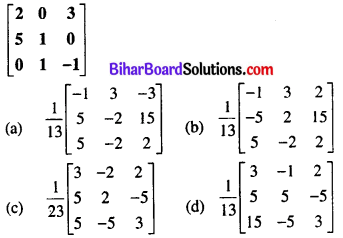(a) $$\frac{1}{13}\left[\begin{array}{ccc} -1 & 3 & -3 \\ 5 & -2 & 15 \\ 5 & -2 & 2 \end{array}\right]$$

Question 30.(c) $$\left[\begin{array}{ccc} 0 & -1 & 1 \\ -4 & 3 & -2 \\ -3 & 3 & -2 \end{array}\right]$$

Question 31.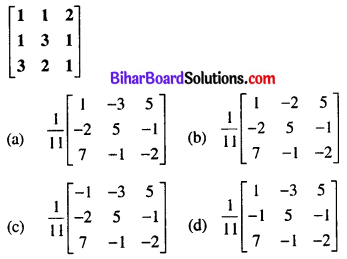(c) $$\frac{1}{11}\left[\begin{array}{ccc} -1 & -3 & 5 \\ -2 & 5 & -1 \\ 7 & -1 & -2 \end{array}\right]$$

Question 32.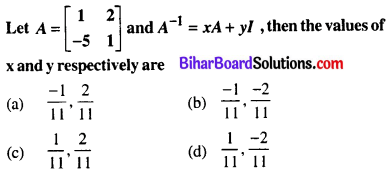(a) $$\frac{-1}{11}, \frac{2}{11}$$

Question 33.
Using elementary transformation, find the inverse of matrix $$\left[\begin{array}{ccc} -1 & 1 & 2 \\ 1 & 2 & 3 \\ 3 & 1 & 1 \end{array}\right]$$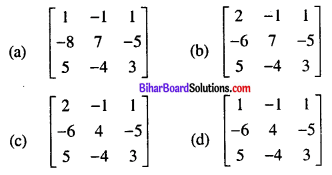(a) $$\left[\begin{array}{ccc} 1 & -1 & 1 \\ -8 & 7 & -5 \\ 5 & -4 & 3 \end{array}\right]$$

Question 34.
Find the inverse of the matrix $$A=\left[\begin{array}{ll} 1 & 3 \\ 2 & 7 \end{array}\right]$$, using elementary row transformation.(a) $$\left[\begin{array}{cc} 7 & -3 \\ -2 & 1 \end{array}\right]$$

Question 35.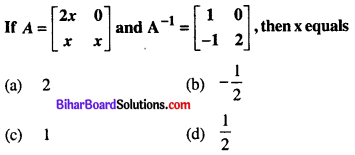(d) $$\frac{1}{2}$$

Question 36.
If A2 – A + I = O, then the inverse of A is
(a) I – A
(b) A – I
(c) A
(d) A + I
(a) I – AQuestion 37.
Total number of possible matrices of order 3 × 3 with each entry 2 or 0 is
(a) 9
(b) 27
(c) 81
(d) 512
(d) 512

Question 38.
The matrix $$\left[\begin{array}{ccc} 0 & -5 & 8 \\ 5 & 0 & 12 \\ -8 & -12 & 0 \end{array}\right]$$ is a
(a) diagonal matrix
(b) symmetric matrix
(c) skew symmetric matrix
(d) scalar matrix
(c) skew symmetric matrix

Question 39.
If A is a matrix of order m × n and B is a matrix such that AB’ and B’A are both defined, then the order of matrix B is
(a) m × m
(b) n × n
(c) n × m
(d) m × n
(d) m × nQuestion 40.
If A and B are matrices of the same order, then (AB’ – BA’) is a
(a) skew-symmetric matrix
(b) null matrix
(c) symmetric matrix
(d) unit matrix